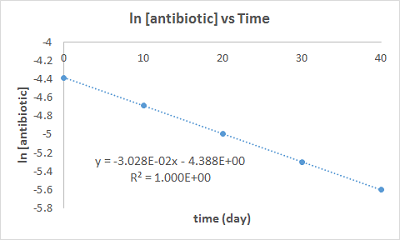A pharmacist must calculate the shelf life for an antibiotic. The antibiotic is stored as a solid...

Question:

A pharmacist must calculate the shelf life for an antibiotic. The antibiotic is stored as a solid and a fresh solution must be prepared for the patient. The antibiotic is unstable in solution and decomposes according to the data in the table below.

{eq}\begin{array}{|c|c|} \hline \text{Time (days)} & \text{[Antibiotic] (Mol/L)} \\ \hline \text{0} & 1.24 \times 10^{-2} \\ \hline \text{10.} & 0.92 \times 10^{-2} \\ \hline \text{20.} & 0.68 \times 10^{-2} \\ \hline \text{30.} & 0.50 \times 10^{-2} \\ \hline \text{40.} & 0.37 \times 10^{-2} \\ \hline \end{array} {/eq}

This is a first order process. Calculate the half-life for the antibiotic. The units should be in days and should be calculated to three significant figures.

If you start with a 1.0 M solution, how long would it take for 77% of the antibiotic to decompose? The answer should be in days and should be calculated to three significant figures.

First Order Kinetics

For first order kinetics, natural log of reactant concentration plotted against time should yield a straight line. The fitted line corresponds to the integrated rate law equation

$$ln [A]_t = -kt + ln [A]_o$$

which allows one to extrac the rate constant (k) of the reaction.

Become a Study.com member to unlock this answer! Create your account

Part : {eq}t_{1/2} = 22.9\;day {/eq}

Part 2: {eq}36.6\;days {/eq}

Part 1

Plotting {eq}ln [antibiotic] \;vs\; t {/eq} is shown below.From...First-Order Reactions: Definition & Mathematical Representation

from

Chapter 19 / Lesson 6
24K

In chemistry, first-order reactions are linear and depend on just one reactant. Explore the definition and mathematical representation of first-order reactions, and review reaction rates, differential rate law, integrated rate law, half-life, and a sample problem to gain understanding.